# C++ Program to Find Quotient and Remainder

In this tutorial you will learn about the C++ Program to Find Quotient and Remainder and its application with practical example.

## C++ Program to Find Quotient and Remainder

In this tutorial, we will learn to create a C++ program that will Find Quotient and Remainder in C++ programming.

## Prerequisites

Before starting with this tutorial we assume that you are best aware of the following C++ programming topics:

• Operators in C++ Programming.
• Basic Input and Output function in C++ Programming.
• Basic C++ Programming.

## What are Quotient and Remainder?

In the divide operation, the remaining part in the operation is a remainder. The answer part we get after the operation is a quotient.

## Program to Find Quotient and Remainder:-

The integer values are used with operators to perform the arithmetic in programming. The divide operation is done in mathematics to get the remainder and quotient value. First, we will take two numbers and divide them to find the quotient and remainder.

With the help of this program, we can take input and Find Quotient and Remainder.

## Output:-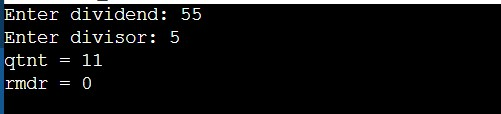In the above program, we have first initialized the required variable.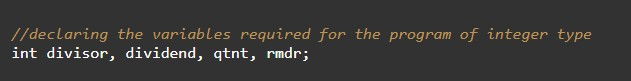• divisor = it will hold the input number value from the user.
• dividend= it will hold the input number value from the user.
• qtnt = it will hold the integer value of the Quotient.
• rmdr = it will hold the integer value of the Remainder.

Taking Input divisor and dividend from the user.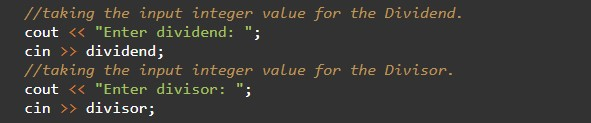Calculating the quotient and the remainder.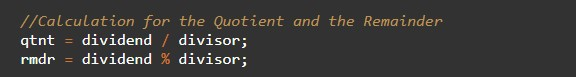Printing the output.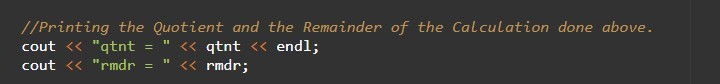In this tutorial we have learn about the C++ Program to Find Quotient and Remainder and its application with practical example. I hope you will like this tutorial.